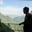Related Tags

devrtt
estimatedrtt
time-out
communitycreator

# How to compute DevRTT, estimated RTT, & time-out interval in CCNAKASH BAJWA

### Overview

We use different methods and mediums for sending and receiving data in our daily life, like SMSShort Message (or Messaging) Service. Similarly, if we talk about computers, communications occur through networks in computers. Imagine that you send a text to your friend and are waiting for their reply, and then you receive a reply. The time that is taken to complete the process of requesting (your text) and receiving a response (friend's answer) is known as RTT (round trip time).

### Measures of RTT

There are three ways to calculate RTT:

1. Estimated RTT
2. Deviation in RTT
3. Time-out Interval

### Estimated RTT

In computer communication networking, the RTTsRound Trip Time of different packets can be different. For example, the first packet takes the round trip time of 1.1ms, the second packet takes 1.3ms, and the third packet takes 0.98ms so, each sample RTT varies. That is why estimated RTT is used, as it is the average of recent measurements, not just the current sample RTT.

#### Formula:

Estimated RTT = (1- α) * Estimated RTT + α * Sample RTT

Where typically, the value of α = 0.125.

For example, the sample RTT is 100ms, we have to compute the estimated RTT using α = 0.125, and we assume the value of the estimated RTT just before the sample RTT was 110ms. So, by using the formula, we get:

Estimated RTT = (1-0.125) * 110ms + (0.125) * 100ms

Estimated RTT = (0.875) * 110ms + 12.5ms

Estimated RTT = 82.5ms + 12.5ms = 95ms

Hence, the required estimated RTT is 95ms.

### Deviation in RTT

Deviation in RTT, also known as Dev-RTT is a measure that indicates how evenly the RTT is distributed during the measurement. It depends upon the previous estimated RTT and helps to find the retransmission time-out.

#### Formula:

DevRTT = (1- β) * DevRTT + β * |Sample RTT - Estimated RTT|

Where typically, the value of β = 0.125.

For example, the sample RTT is 100ms, we have to compute the DevRTT, and we assume the value of the estimated RTT is 95ms and the previous DevRTT was 20ms. So, by using the formula, we get:

Dev RTT = (1-0.25) * 20ms + (0.25) * |100ms - 95ms|

Dev RTT = (0.75) * 20ms + (0.25) * 5ms

Dev RTT = 15ms + 1.5ms = 16.5ms

Hence, the required DevRTT is 16.5ms.

### Time-out

Time-out is longer than RTT, but as RTT varies, we need to add some margin i.e., safety margin, to it. The selection of a time-out value is essential because the longer the value of estimated RTT, the slower its performance. We will be facing long delays in this case. Similarly, in the case of a minimal value, the connection can be lost before the RTT completes or before the arrival of the response or acknowledgment. So for the safety, we use:

#### Formula:

Time-out Interval = 4 * DevRTT + Estimated RTT

Where the DevRTT value is used for a safety margin.

So, using the above-computed values of DevRTT = 16.5ms and Estimated RTT = 95ms, we compute the time-out interval as:

Time-out Interval = 4 * 16.5ms + 95ms

Time-out Interval = 66ms + 95ms = 161ms

Hence, the required time-out interval is 161ms.

We can see that the time-out interval is dependent on both DevRTT and estimated RTT. Also, DevRTT is dependent on estimated RTT. So, if we need to find DevRTT, we have to compute the estimated RTT first. To compute the time-out interval, we have to find both DevRTT and estimated RTT first.

RELATED TAGS

devrtt
estimatedrtt
time-out
communitycreator

CONTRIBUTORAKASH BAJWA
RELATED COURSES

View all Courses

Keep Exploring

Learn in-demand tech skills in half the time